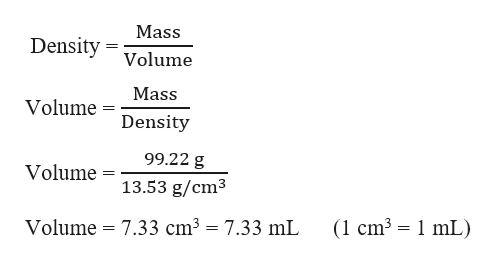An empty vial weighs 59.43 g. If the vial weighs 158.65g when filled with liquid mercury (d=13.53 g/cm^3), what is the volume of mercury?

Question

An empty vial weighs 59.43 g. If the vial weighs 158.65g when filled with liquid mercury (d=13.53 g/cm^3), what is the volume of mercury?

Step 1

Given,

Mass of empty vial = 59.43 g

Mass of vial filled with liquid mercury = 158.65 g

Mass of liquid mercury = 158.65 g - 59.43 g   = 99....help_outlineImage TranscriptioncloseMass Density Volume Mass Volume Density 99.22 g Volume 13.53 g/cm3 (1 cm3 1 mL) = 7.33 cm3 = 7.33 mL Volume fullscreen

Want to see the full answer?

See Solution

Want to see this answer and more?

Our solutions are written by experts, many with advanced degrees, and available 24/7

See Solution
Tagged in

General Chemistry